• CVXOPT 用于凸优化的Python软件 发布信息 相关项目 建造状态C
• cvxopt-1.1.9-cp27-cp27m-win_amd64.whl 使用安装文章地址：https://blog.csdn.net/qq_36477513/article/details/104779850cvxopt
• 支持向量机 无需sklearn即可从头开始进行教育性SVM实现。 CVXOPT用作方程式求解器。 对于二进制分类器，标签应为[-1，1]。 multi_SVM.py是使用OneVsRest策略的多类SVM。 支持自定义内核，实现了线性和rbf内核。
• cvxopt-1.1.9-cp34-cp34m-win_amd64数据
• cvxopt-1.2.3-cp37-cp37m-win_amd64.whl ，原地址太慢了，自己在csdn上做个备份 原地址：https://www.lfd.uci.edu/~gohlke/pythonlibs/#cvxopt
• 主要介绍了Python CVXOPT模块安装及使用解析,文中通过示例代码介绍的非常详细，对大家的学习或者工作具有一定的参考学习价值,需要的朋友可以参考下
• cvxopt

2019-03-30 21:28:53
cvxopt是python上求解最优化问题的一个包，在数值计算、规划问题和运筹中都有广泛应用。

cvxopt是python上求解最优化问题的一个包，在数值计算、规划问题和运筹中都有广泛应用。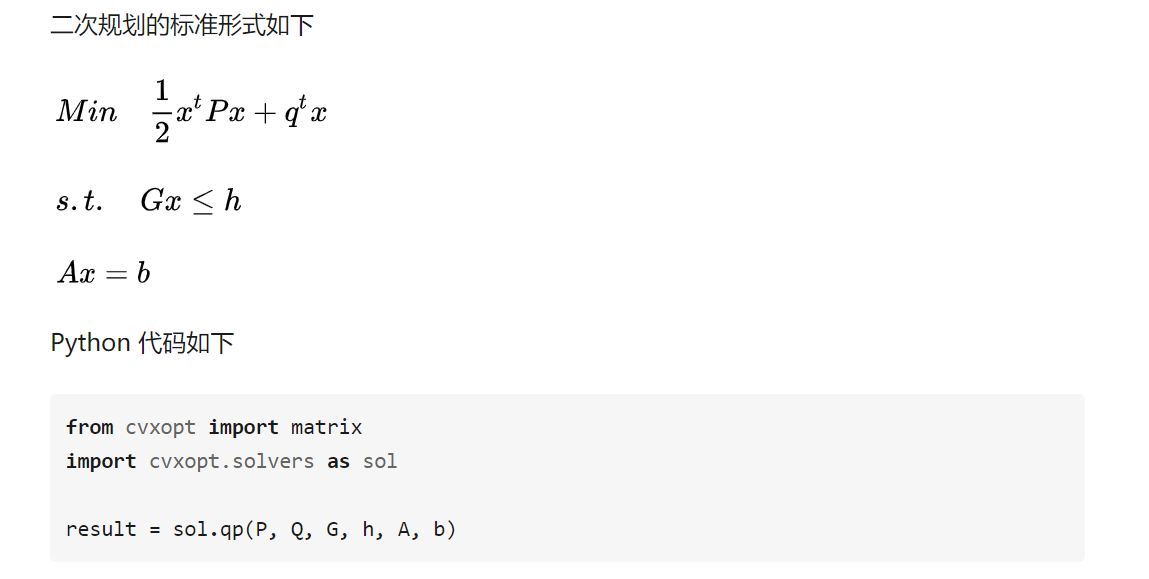参见

注：

在python27上安装了cvxopt之后，import numpy出错：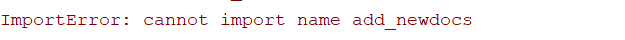解决方法是在python命令窗口输入 “conda install numpy” ：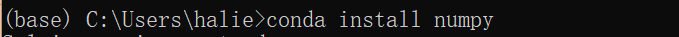其他的如pandas不能用，也用如上办法。

展开全文• CVXOPT 网站提供的文档： http ://www.cvxopt.orgmatlab
• cvxopt-1.2.4-cp37-cp37m-manylinux1_x86_64.whlpython
• cvxopt-1.2.6-cp39-cp39-win32数据
• cvxopt-1.2.3-cp35-cp35m-win32数据
• 源来源：https://www.lfd.uci.edu/~gohlke/pythonlibs/#...本版本兼容性好，可以直接安装cvxopt，详细说明见我的博客 版本匹配： numpy-1.16.3+mkl-cp36-cp36m-win_amd64.whl cvxopt-1.2.3-cp36-cp36m-win_amd64.whl
• cs229-cvxopt2.pdf

2019-08-21 16:30:53Delphi
• Py之cvxoptcvxopt库的简介、安装、使用方法之详细攻略 目录 cvxopt库的简介 cvxopt库的安装 cvxopt库的使用方法 1、创建矩阵 2、求解线性规划 cvxopt库的简介 CVXOPT是一个基于Python编程语言...

Py之cvxopt：cvxopt库的简介、安装、使用方法之详细攻略

目录

cvxopt库的简介

cvxopt库的安装

cvxopt库的使用方法

1、创建矩阵

2、求解线性规划

cvxopt库的简介

CVXOPT是一个基于Python编程语言的凸优化的免费软件包。它可以与交互式Python解释器一起使用，也可以通过执行Python脚本在命令行上使用，或者通过Python扩展模块集成到其他软件中。它的主要目的是通过构建Python的广泛标准库和Python作为一种高级编程语言的优势，使凸优化应用程序的软件开发变得简单。

cvxopt库的安装

pip install cvxopt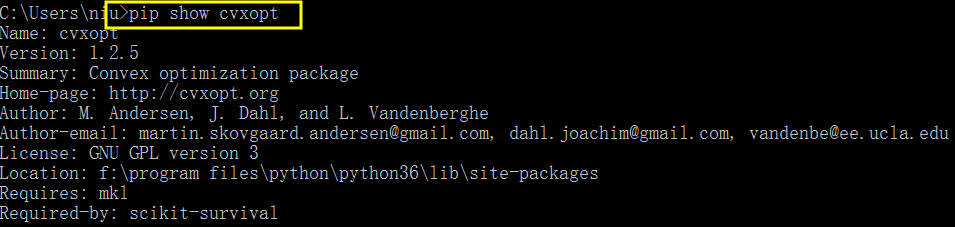cvxopt库的使用方法

1、创建矩阵

CVXOPT有单独的稠密和稀疏矩阵对象。这个例子演示了创建密集和稀疏矩阵的不同方法。使用matrix()函数创建一个密集矩阵;它可以通过列表(或迭代器)创建:

>>> from cvxopt import matrix
>>> A = matrix([1.0, 2.0, 3.0, 4.0, 5.0, 6.0], (2,3))
>>> print(A)
[ 1.00e+00  3.00e+00  5.00e+00]
[ 2.00e+00  4.00e+00  6.00e+00]
>>> A.size
(2, 3)

>>> B = matrix([ [1.0, 2.0], [3.0, 4.0] ])
>>> print(B)
[ 1.00e+00  3.00e+00]
[ 2.00e+00  4.00e+00]

>>> print(matrix([ [A] ,[B] ]))
[ 1.00e+00  3.00e+00  5.00e+00  1.00e+00  3.00e+00]
[ 2.00e+00  4.00e+00  6.00e+00  2.00e+00  4.00e+00]

>>> from cvxopt import spmatrix
>>> D = spmatrix([1., 2.], [0, 1], [0, 1], (4,2))
>>> print(D)
[ 1.00e+00     0    ]
[    0      2.00e+00]
[    0         0    ]
[    0         0    ]
>>> print(matrix(D))
[ 1.00e+00  0.00e+00]
[ 0.00e+00  2.00e+00]
[ 0.00e+00  0.00e+00]
[ 0.00e+00  0.00e+00]

2、求解线性规划

可以通过求解器.lp()函数指定线性程序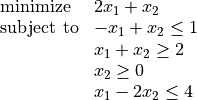>>> from cvxopt import matrix, solvers
>>> A = matrix([ [-1.0, -1.0, 0.0, 1.0], [1.0, -1.0, -1.0, -2.0] ])
>>> b = matrix([ 1.0, -2.0, 0.0, 4.0 ])
>>> c = matrix([ 2.0, 1.0 ])
>>> sol=solvers.lp(c,A,b)
pcost       dcost       gap    pres   dres   k/t
0:  2.6471e+00 -7.0588e-01  2e+01  8e-01  2e+00  1e+00
1:  3.0726e+00  2.8437e+00  1e+00  1e-01  2e-01  3e-01
2:  2.4891e+00  2.4808e+00  1e-01  1e-02  2e-02  5e-02
3:  2.4999e+00  2.4998e+00  1e-03  1e-04  2e-04  5e-04
4:  2.5000e+00  2.5000e+00  1e-05  1e-06  2e-06  5e-06
5:  2.5000e+00  2.5000e+00  1e-07  1e-08  2e-08  5e-08
>>> print(sol['x'])
[ 5.00e-01]
[ 1.50e+00]

展开全文• Qp-cvxopt.pdf

2019-05-17 20:37:06
Python 中做线性规划和二次规划的包，案例说明和具体参数说明，中文资源很少
• 64位win平台下python3.6的cvxopt包。包含numpy+mkl包和cvxopt包。凸二次规划
• 优化问题优化问题无约束优化Nelder-Mead 单纯形法Broyden-Fletcher-Goldfarb-Shanno牛顿共轭梯度法有约束优化问题CVXOPT 解 二次规划问题 import numpy as np import scipy.optimize as opt 优化问题 这里讨论的...

看 Python 量化金融投资，摘录的一些统计函数。为了以后更好的查找。

import numpy as np
import scipy.optimize as opt

优化问题

• 这里讨论的问题全部是凸优化问题，即目标函数为凸函数，其自变量的可行集为凸集（详细定义可见 斯坦福大学教材 剑桥课件

无约束优化

• 无约束优化问题是指一个优化问题的寻优可行集合是目标函数自变量的定义域，即没有外部的限制条件。

• 例如，求解优化问题：

• Minimize  f ( x ) = x 2 − 4.8 x + 1.2 \text{Minimize } f(x) = x^2 - 4.8x + 1.2
• 为无约束优化问题
• 转为带约束的优化问题

• min  f ( x ) = x 2 − 4.8 x + 1.2 \text{min } f(x) = x^2 - 4.8x + 1.2
• s.t  x ≥ 0 \text{s.t } x\geq 0
• Rosenbrock 函数举例
f ( x ) = ∑ i = 1 N − 1 100 ( x i − x i − 1 2 ) 2 + ( 1 − x i − 1 ) 2 f(x) = \sum^{N-1}_{i=1}100(x_i-x_{i-1}^2 )^2+(1-x_{i-1})^2

# Rosenbrock
def rosen(x):
"""
The Rosenbrock Function
"""
return sum(100.0*(x[1:] - x[:-1]**2.)**2.+(1-x[:-1])**2.)

• 设定起始点 x 0 = [ 0.5 , 1.6 , 1.1 , 0.8 , 1.2 ] x_0 = [0.5,1.6,1.1,0.8,1.2] 并行性最小化寻优。
• xtol 表示迭代收敛的容忍误差上界。
• 其全域最小值位于 x i = 1 x_i=1 , 数值为 f ( x i ) = 0 f(x_i)=0
x_0 = np.array([0.5,1.6,1.1,0.8,1.2])

Optimization terminated successfully.
Current function value: 0.000000
Iterations: 386
Function evaluations: 622

final_simplex: (array([[1.        , 1.00000003, 1.00000007, 1.00000017, 1.0000003 ],
[1.        , 0.99999999, 1.00000004, 1.0000001 , 1.00000022],
[0.99999999, 0.99999999, 1.00000004, 1.00000007, 1.00000011],
[0.99999998, 0.99999993, 0.99999991, 0.99999982, 0.9999996 ],
[1.00000008, 1.00000016, 1.00000033, 1.00000067, 1.00000127],
[1.00000002, 1.00000004, 1.00000015, 1.00000033, 1.0000006 ]]), array([4.14390143e-13, 4.94153342e-13, 5.19631652e-13, 5.35708759e-13,
9.80969042e-13, 9.81943884e-13]))
fun: 4.143901431865225e-13
message: 'Optimization terminated successfully.'
nfev: 622
nit: 386
status: 0
success: True
x: array([1.        , 1.00000003, 1.00000007, 1.00000017, 1.0000003 ])

• Rosenbrock 函数性能比较好，简单的优化方法就可以处理了。
• 还可以使用 Powell 方法，method="powell"
opt.minimize(rosen,x_0,method='powell',options={'xtol':1e-6,'disp':True})

Optimization terminated successfully.
Current function value: 0.000000
Iterations: 24
Function evaluations: 1618

direc: array([[ 4.55588497e-04,  1.36409332e-03,  2.24683410e-03,
4.12376042e-03,  7.99776305e-03],
[-1.91331747e-03, -3.00268845e-03, -6.76968505e-03,
-1.34778007e-02, -2.66903472e-02],
[-3.76306326e-02, -2.30543912e-02,  1.05016733e-02,
3.42182501e-05,  7.33576548e-05],
[ 0.00000000e+00,  0.00000000e+00,  0.00000000e+00,
1.00000000e+00,  0.00000000e+00],
[ 4.02021587e-06,  1.15777807e-05,  2.01895943e-05,
5.10192097e-05,  1.09407425e-04]])
fun: 2.051360957724131e-21
message: 'Optimization terminated successfully.'
nfev: 1618
nit: 24
status: 0
success: True
x: array([1., 1., 1., 1., 1.])

• 这两种方法并不使用函数的梯度，在略微复杂的情形下收敛速度比较慢。接下来介绍 利用函数梯度寻优。

Broyden-Fletcher-Goldfarb-Shanno

• Broyden-Fletcher-Goldfarb-Shanno(BFGS) 修正拟牛顿法

• 首先求 Rosenbrock 函数的梯度：

∂ f ∂ x j = ∑ i = 1 N 200 ( x i − x i − 1 ) + ( δ i , j − 2 x i − 1 δ i − 1 , j ) = 200 ( x j − x j − 1 2 ) − 400 x j ( x j + 1 − x j 2 ) − 2 ( 1 − x j ) \begin{aligned}\frac{\partial f}{\partial x_j} &= \sum^{N}_{i=1}200(x_i-x_{i-1})+(\delta_{i,j}-2 x_{i-1}\delta_{i-1,j})\\&=200(x_j -x_{j-1}^2)-400x_j(x_{j+1}-x_j^2)-2(1-x_j)\end{aligned}

• { δ i , j = 1 i = j δ i , j = 0 else \begin{aligned}\begin{cases}\delta_{i,j}=1 & i=j \\ \delta_{i,j} =0 &\text{else}\end{cases}\end{aligned}
• 特例:
• ∂ f ∂ x 0 = − 400 x 0 ( x 1 − x 0 2 ) − 2 ( 1 − x 0 ) \frac{\partial f}{\partial x_0} = -400 x_0(x_1-x_0^2)-2(1-x_0)
• ∂ f ∂ x N − 1 = 200 ( x N − x N − 1 2 ) \frac{\partial f}{\partial x_{N-1}} = 200(x_{N}-x^2_{N-1})
def rosen_der(x):
xj = x[1:-1]
xj_m1 = x[:-2]
xj_p1 = x[2:]
der = np.zeros_like(x)
der[1:-1] = 200*(xj-xj_m1**2) -400*xj*(xj_p1-xj**2)-2*(1-xj)
der = -400*x*(x-x**2)-2*(1-x)
der[-1] = 200*(x[-1]-x[-2]**2)
return der

opt.minimize(rosen,x_0,method='BFGS',jac=rosen_der,options={'disp':True})

Optimization terminated successfully.
Current function value: 0.000000
Iterations: 39
Function evaluations: 47

fun: 1.569191726013783e-14
hess_inv: array([[0.00742883, 0.01251316, 0.02376685, 0.04697638, 0.09387584],
[0.01251316, 0.02505532, 0.04784533, 0.094432  , 0.18862433],
[0.02376685, 0.04784533, 0.09594869, 0.18938093, 0.37814437],
[0.04697638, 0.094432  , 0.18938093, 0.37864606, 0.7559884 ],
[0.09387584, 0.18862433, 0.37814437, 0.7559884 , 1.51454413]])
jac: array([-3.60424798e-06,  2.74743159e-06, -1.94696995e-07,  2.78416205e-06,
-1.40985001e-06])
message: 'Optimization terminated successfully.'
nfev: 47
nit: 39
njev: 47
status: 0
success: True
x: array([1.        , 1.00000001, 1.00000002, 1.00000004, 1.00000007])

• 这里 梯度信息的引入 在 minimize 函数中通过 jac 指定。
• 可以看到迭代次数比之前小了不少。
• Iterations 迭代次数
• Function evaluations 函数调用次数

牛顿共轭梯度法

• 简称 牛顿法
• 收敛速度最快的方法，缺点在于求解 Hessian 矩阵（二阶导数矩阵）
• 大致思路：采用泰勒展开的二阶近似，使用共轭梯度近似 Hessian 矩阵的逆矩阵。
• Rosenbrock 函数 的 Hessian 矩阵元素通式：
H ( i , j ) = ∂ 2 f ∂ x i ∂ x j = 200 ( δ i , j − 2 x i − 1 δ i − 1 , j ) − 400 x i ( δ i + 1 , j − 2 x i δ i , j ) − 400 δ i , j ( x i + 1 , j − x i 2 ) + 2 δ i , j H(i,j) = \frac{\partial^2 f}{\partial x_i\partial x_j} = 200(\delta_{i,j}-2x_{i-1}\delta_{i-1,j})-400x_i(\delta_{i+1,j}-2x_i\delta_{i,j})-400\delta_{i,j}(x_{i+1,j}-x_i^2)+2\delta_{i,j}

H ( i , j ) = ( 202 + 1200 x i 2 − 400 x i + 1 ) δ i , j − 400 x i δ i + 1 , j − 400 x i − 1 δ i − 1 , j H(i,j) = (202+1200x_i^2-400x_{i+1})\delta_{i,j}-400x_i\delta_{i+1,j}-400x_{i-1}\delta_{i-1,j}

• 边界条件：
• ∂ 2 f ∂ x 0 2 = 1200 x 0 2 − 400 x 1 + 2 \frac{\partial^2 f}{\partial x_0^2} = 1200x_0^2 -400 x_1 +2
• ∂ 2 f ∂ x 0 ∂ x 1 = − 400 x 0 \frac{\partial^2 f}{\partial x_0\partial x_1} = -400x_0
• ∂ 2 f ∂ x N − 1 ∂ x N − 2 = − 400 x N − 2 \frac{\partial^2 f}{\partial x_{N-1}\partial x_{N-2}} = -400x_{N-2}
• ∂ 2 f ∂ x N − 1 2 = 200 \frac{\partial^2 f}{\partial x_{N-1}^2} = 200
def rosen_hess(x):
H = np.diag(-400*x[:-1],1)+np.diag(-400*x[:-1],-1)
diagonal = np.zeros_like(x)
diagonal = 1200*x**2 -400*x+2
diagonal[-1] = 200
diagonal[1:-1] = 202+1200*x[1:-1]**2 -400*x[2:]
H = H+np.diag(diagonal)
return H

opt.minimize(rosen,x_0,method='Newton-CG',jac=rosen_der,hess=rosen_hess,options={'xtol':1e-6,'disp':True})

Optimization terminated successfully.
Current function value: 0.000000
Iterations: 20
Function evaluations: 22
Hessian evaluations: 20

fun: 1.47606641102778e-19
jac: array([-3.62847530e-11,  2.68148992e-09,  1.16637362e-08,  4.81693414e-08,
-2.76999090e-08])
message: 'Optimization terminated successfully.'
nfev: 22
nhev: 20
nit: 20
njev: 41
status: 0
success: True
x: array([1., 1., 1., 1., 1.])

• 对于一些大型的优化问题，Hessian 矩阵将异常大，牛顿法用到的仅是Hessian 矩阵的一个任意向量的乘积。
• 因此只要提供一个向量p，就减少存储的开销。
def rosen_hessp(x,p):
Hp = np.zeros_like(x)
Hp = (1200*x**2-400*x+2)*p -400*x*p
Hp[1:-1] = -400*x[:-2]*p[:-2]+(202+1200*x[1:-1]**2-400*x[2:])*p[1:-1]-400*x[1:-1]*p[2:]
Hp[-1] = -400*x[-2]*p[-2]+200*p[-1]
return Hp

opt.minimize(rosen,x_0,method='Newton-CG',jac=rosen_der,hessp=rosen_hessp,options={'xtol':1e-6,'disp':True})

Optimization terminated successfully.
Current function value: 0.000000
Iterations: 20
Function evaluations: 22
Hessian evaluations: 58

fun: 1.47606641102778e-19
jac: array([-3.62847530e-11,  2.68148992e-09,  1.16637362e-08,  4.81693414e-08,
-2.76999090e-08])
message: 'Optimization terminated successfully.'
nfev: 22
nhev: 58
nit: 20
njev: 41
status: 0
success: True
x: array([1., 1., 1., 1., 1.])

有约束优化问题

• 标准形式为：
min  f ( x ) s.t.  g i ( x ) ≤ 0 ,   i = 1 , 2 , ⋯   , m A x = b \begin{aligned}\text{min } &f(x)\\\text{s.t. }&g_i(x)\leq0, \ i=1,2,\cdots, m\\ Ax&=b\end{aligned}

• 其中 g 1 , ⋯   , g m : R n → R g_1,\cdots, g_m: \mathbb{R}^n\to \mathbb{R} R n \mathbb{R}^n 空间上的二次可微分的凸函数； A A p × n p\times n 矩阵且秩 rank ( A ) = p < n \text{rank}(A)=p< n

• 例：
Minimize  f ( x , y ) = 2 x y + 2 x − x 2 − 2 y 2 subject to  x 3 − y = 0 y − 1 ≥ 0 \begin{aligned}\text{Minimize } &f(x,y) = 2xy+2x-x^2-2y^2\\\text{subject to }& x^3-y = 0\\ y-1\geq0\end{aligned}

def func(x,sign=1.):
"""
objective function
"""
return sign*(2*x*x+2*x -x**2-2*x**2)

def func_deriv(x,sign=1.):
"""
derivative of objective function
"""
dfdx0 = sign * (-2*x+2*x+2)
dfdx1 = sign * (-4*x+2*x)
return np.array([dfdx0,dfdx1])

• 其中 sign 表示求解 最大或最小值。进一步定义约束条件：
cons = ({'type':'eq', 'fun':lambda x:np.array([x**3. -x]),\
'jac':lambda x: np.array([3.*x**2.,-1.])},\
{'type':'ineq','fun':lambda x: np.array([x-1]),\
'jac':lambda x: np.array([0,1])})

• 最后使用 SLSQP (sequential Least SQuares Programming optimization algorithm)
opt.minimize(func,[-1.,1.],\
args=(-1.,),\
jac=func_deriv, \
constraints=cons,method='SLSQP',\
options={'disp':True})

Optimization terminated successfully.    (Exit mode 0)
Current function value: -1.0000001831052137
Iterations: 9
Function evaluations: 14

fun: -1.0000001831052137
jac: array([-1.99999982,  1.99999982])
message: 'Optimization terminated successfully.'
nfev: 14
nit: 9
njev: 9
status: 0
success: True
x: array([1.00000009, 1.        ])

print('无约束优化进行比较')
print('Result of unconstrained optimization')
opt.minimize(func,[-1.,1.],\
args=(-1.,),\
jac=func_deriv, \
method='SLSQP',\
options={'disp':True})

无约束优化进行比较
Result of unconstrained optimization
Optimization terminated successfully.    (Exit mode 0)
Current function value: -2.0
Iterations: 4
Function evaluations: 5

fun: -2.0
jac: array([-0., -0.])
message: 'Optimization terminated successfully.'
nfev: 5
nit: 4
njev: 4
status: 0
success: True
x: array([2., 1.])

CVXOPT 解 二次规划问题

• Python 中 除了可以使用 scipy.minimize 工具处理优化问题以外，也有专门处理优化的扩展模块，例如 CVXOPT
• 二次规划问题标准形式：

min  1 2 x T P x + q T x s.t.  G x ≤ h ,   A x = b \begin{aligned}\text{min } &\frac{1}{2} x^T P x + q^T x\\\text{s.t. }&Gx\leq h, \ Ax=b\end{aligned}

• 例： 求：
min  2 x 2 + x y + y 2 + x + y s.t.  x ≥ 0 ,   y ≥ 0 x + y = 1 \begin{aligned}\text{min } &2x^2 + xy + y^2 + x + y\\ \text{s.t. } &x\geq 0,\ y\geq 0\\& x+y=1\end{aligned}

p = [ 4 1 1 2 ] q = [ 1 1 ] G = [ − 1 0 0 − 1 ] ⋯ \begin{aligned} p &= \begin{bmatrix} 4 & 1 \\ 1 & 2 \end{bmatrix}\\ q &= \begin{bmatrix} 1 & 1\end{bmatrix}\\ G &= \begin{bmatrix} -1 & 0 \\ 0 & -1 \end{bmatrix}\\ \cdots\end{aligned}

from cvxopt import solvers,matrix

p = matrix([[4., 1.], [1., 2.]])
q = matrix([1., 1.])
G = matrix([[-1.,0.],[0.,-1.]])
h = matrix([0.,0.]) # matrix里区分int和double，所以数字后面都需要加小数点
A = matrix([1., 1.], (1,2)) # A必须是一个1行2列
b = matrix(1.)

sol=solvers.qp(p, q, G, h, A, b)
print(sol['x'])

pcost       dcost       gap    pres   dres
0:  1.8889e+00  7.7778e-01  1e+00  3e-16  2e+00
1:  1.8769e+00  1.8320e+00  4e-02  2e-16  6e-02
2:  1.8750e+00  1.8739e+00  1e-03  2e-16  5e-04
3:  1.8750e+00  1.8750e+00  1e-05  6e-17  5e-06
4:  1.8750e+00  1.8750e+00  1e-07  2e-16  5e-08
Optimal solution found.
[ 2.50e-01]
[ 7.50e-01]

• 解得 x = 0.25 , y = 0.75 x=0.25,y=0.75
• 以下是 solvers 内的其他参数：
sol

{'x': <2x1 matrix, tc='d'>,
'y': <1x1 matrix, tc='d'>,
's': <2x1 matrix, tc='d'>,
'z': <2x1 matrix, tc='d'>,
'status': 'optimal',
'gap': 1.0527028380515569e-07,
'relative gap': 5.6144154514915067e-08,
'primal objective': 1.8750000000000182,
'dual objective': 1.8749998947297344,
'primal infeasibility': 2.482534153247273e-16,
'dual infeasibility': 5.3147593337403756e-08,
'primal slack': 0.2500000952702474,
'dual slack': 1.0000000000000035e-08,
'iterations': 4}

投资组合中的应用

• 给出下列3个资产数据
• s1 , s2 , b
s1 = [0.,.04,.13,.19,-.15,-.27,.37,.24,-.07,.07,.19,.33,-.05,.22,.23,.06,.32,.19,.05,.17]
s2 = [.07,.13,.14,.43,.67,.64,0.,-.22,.18,.31,.59,.99,-.25,.04,-.11,-.15,-.12,.16,.22,-.02]
b = [.06,.07,.05,.04,.07,.08,.06,.04,.05,.07,.1,.11,.15,.11,.09,.1,.08,.06,.05,.07]
x = np.array([s1,s2,b]) # 资产数据
p = matrix(np.cov(x)) # 求得协方差矩阵
q = matrix([0.,0.,0.])
A = matrix([[1.],[1.],[1.]])
b = matrix([1.])
G = matrix([[-1.,0.,0.,1.,0.,0.,-.113],\
[0.,-1.,0.,0.,1.,0.,-.185],\
[0.,0.,-1.,0.,0.,1.,-.0755]])
h = matrix([0.,0.,0.,1.,1.,1.,-.13])
sol = solvers.qp(p,q,G,h,A,b)

pcost       dcost       gap    pres   dres
0:  6.1729e-03 -3.3446e+00  1e+01  2e+00  4e-01
1:  7.4918e-03 -1.5175e+00  2e+00  2e-02  5e-03
2:  9.0497e-03 -7.7600e-02  9e-02  1e-03  3e-04
3:  8.4511e-03  2.0141e-03  6e-03  7e-05  2e-05
4:  7.6044e-03  7.1661e-03  4e-04  3e-07  8e-08
5:  7.5351e-03  7.5293e-03  6e-06  3e-09  8e-10
6:  7.5340e-03  7.5340e-03  6e-08  3e-11  8e-12
Optimal solution found.

print(sol['x'])

[ 5.06e-01]
[ 3.24e-01]
[ 1.69e-01]

• 可见资产1 投资比例为 51%，资产2 投资比例为 32.4%, 资产3 投资比例为 16.9%
sol

{'x': <3x1 matrix, tc='d'>,
'y': <1x1 matrix, tc='d'>,
's': <7x1 matrix, tc='d'>,
'z': <7x1 matrix, tc='d'>,
'status': 'optimal',
'gap': 5.750887504859599e-08,
'relative gap': 7.633272007468671e-06,
'primal objective': 0.007534031792304567,
'dual objective': 0.007533974289443271,
'primal infeasibility': 3.318376136551126e-11,
'dual infeasibility': 8.328707321687642e-12,
'primal slack': 3.811267544311688e-08,
'dual slack': 1.88724298640649e-09,
'iterations': 6}

展开全文python scipy cvxopt 线性代数
• python实现svmCVXOPT is a free python package that is widely used in solving the convex optimization ... In this article, I will first introduce the use of CVXOPT in quadratic programming, and then ...

python实现svm

CVXOPT is a free python package that is widely used in solving the convex optimization problem. In this article, I will first introduce the use of CVXOPT in quadratic programming, and then discuss its application in implementing Support Vector Machine (SVM) by solving the dual optimization problem.

CVXOPT是一个免费的python软件包，广泛用于解决凸优化问题。 在本文中，我将首先介绍CVXOPT在二次编程中的用途，然后通过解决双重优化问题来讨论其在实现支持向量机(SVM)中的应用。

如何使用CVXOPT解决优化问题(How to use CVXOPT to solve an optimization problem)

To understand how to use CVXOPT, we need to know its standard form and apply it to each individual question. According to CVXOPT API, we can solve the optimization problem in this form:

要了解如何使用CVXOPT，我们需要了解其标准格式并将其应用于每个单独的问题。 根据CVXOPT API，我们可以通过以下形式解决优化问题：

It is solving a minimization problem, with two types of linear constraints. One is an inequality constraint, and another is an equality constraint. To use the package to solve for the best x, that minimizing the object function, under the linear constraints, we just need to transform the specific question to identify matrics P, q, G, h, A, b.

它正在解决具有两种线性约束的最小化问题。 一个是不平等约束，另一个是平等约束。 为了使用包来求解最佳x，从而在线性约束下最小化目标函数，我们只需要变换特定问题即可识别矩阵P，q，G，h，A，b。

Let’s take a simple example from here:

让我们从这里举一个简单的例子：

In this example, we have two variables we need to solve for optimization, which is x1 and x2. First, look at the objective function 2x1² +x2² +x1x2+x1+x2, we can rewrite it as its matrix form:

在此示例中，我们需要求解两个变量以进行优化，分别是x1和x2。 首先，查看目标函数2x1²+x2²+ x1x2 + x1 + x2，我们可以将其重写为矩阵形式：

P is the matrix that captures the two-dimensions’ coefficients. Thus we look at the coefficients of x1², x2², and x1x2 to construct P. Taking into consideration the 1/2 in front, P is:

P是捕获二维系数的矩阵。 因此，我们看一下x1²，x2²和x1x2的系数来构造P。考虑到前面的1/2，P为：

And q is the matrix for x1 and x2, which is:

q是x1和x2的矩阵，即：

The next step is to build the linear constraints, lets start with the inequality to find out G and h. The standard form has equality with the less-than sign, while in the question, we have the larger-than sign. Thus, we need to transform the question by multiply negative one on both sides, we will get:

下一步是建立线性约束，让我们从不等式开始找出G和h。 标准形式与小于号相等，而在问题中，我们具有大于号。 因此，我们需要通过在两边都乘以负数来变换问题，我们将得到：

-x1 ≤ 0

-x1≤0

-x2 ≤ 0

-x2≤0

The corresponding G and h is:

相应的G和h为：

And A, b is straightforward:

A，b很简单：

A is a diagonal matrix and b is a scalar.

A是对角矩阵，b是标量。

With this example, I illustrate how we can transform a practical question to match the standard form of the CVXOPT package and find all the matrice to solve the optimization problem.

在此示例中，我说明了如何转换一个实际问题以匹配CVXOPT软件包的标准形式，并找到所有矩阵来解决优化问题。

在SVM上的应用(The application on SVM)

One application of using the CVXOPT package from python is to implement SVM from scratch. Support Vector Machine is a supervised machine learning algorithm that is usually used for binary classification problems, although it is also possible to use it to solve multi-classification problems and regression problems. We define the cost function of the soft margin binary class linear SVM as:

使用来自python的CVXOPT软件包的一个应用是从头开始实现SVM。 支持向量机是一种有监督的机器学习算法，通常用于二进制分类问题，尽管也可以使用它来解决多分类问题和回归问题。 我们将软裕度二进制类别线性SVM的成本函数定义为：

This is the primal problem. The soft margin means that we are allowing some support vectors to across the hyperplane and be assigned to the wrong classes when finding the maximal margin.

这是首要问题。 软边距意味着我们允许某些支持向量穿过超平面，并在找到最大边距时被分配给错误的类。

The support vectors that cross the hyperplane is called slacks. C is a constant, which is a hyper-parameter that defines the “cost” of the slacks. When C is small, it is efficient to allow more points into the margin to achieve a larger margin. Larger C will produce boundaries with fewer support vectors. By increasing the number of support vectors, SVM reduces its variance since it depends less on any individual observation. Reducing variance makes the model more generalized. Thus, decreasing C will increase the number of support vectors and reduce over-fitting.

穿过超平面的支持向量称为松弛。 C是一个常数，它是定义松弛的“成本”的超参数。 当C较小时，允许有更多点进入边距以实现更大的边距是有效的。 C越大，边界越少，支持向量也越少。 通过增加支持向量的数量，SVM减少了方差，因为它较少依赖于任何单独的观察。 减少方差使模型更通用。 因此，降低C将增加支持向量的数量并减少过度拟合。

In solving the primal problem, we are minimizing the cost function regarding both w and b. We can rewrite the constrained optimization problem as the primal Lagrangian function with Lagrange multipliers \alpha_i ≥0 and \mu_i ≥ 0 for our two constraints, and get the following:

在解决原始问题时，我们将w和b的成本函数最小化。 对于两个约束，我们可以用拉格朗日乘数\ alpha_i≥0和\ mu_i≥0将约束优化问题重写为原始拉格朗日函数，并得到以下结果：

Instead of minimizing over w and b, subject to constraints involving \alpha, we can maximize over \alpha subject to the relations obtained previously for w and b. This is called the dual Lagrangian formulation:

代替最小化w和b，受涉及\ alpha的约束，我们可以最大化\ alpha受先前为w和b获得的关系。 这称为双重拉格朗日公式：

Where x are the features and y is the target value. y is defined as 1 for the positive class and -1 for the negative class. In this article, we will show the soft margin implementation of binary class linear SVM by solving the dual problem.

其中x是特征，y是目标值。 y被定义为1(对于正类)和-1(对于负类)。 在本文中，我们将通过解决对偶问题来说明二进制类线性SVM的软边距实现。

First, we need to rewrite the objective function from maximizing to minimizing and rewrite the linear constraints to fit the CVXOPT package. Let’s define a matrix H, which equals to:

首先，我们需要从最大化到最小化重写目标函数，并重写线性约束以适合CVXOPT软件包。 让我们定义一个矩阵H，它等于：

We can rewrite the optimizing problem as:

我们可以将优化问题重写为：

times -1:

-1：

From here, it is clear what are P, q, G, h, A, and b. Suppose we have m features, and n observations. P is the same as H with size equals to m*m; q is a m*1 vector of -1s; G vertically combines two m*m diagonal matrics. The top diagonal value is -1 and the bottom diagonal value is 1. h is a 2m*1 vector with m zeros on the top, and m Cs on the bottom. A is the target value vector y transposed and b is a scaler that equals to 0. Here is a python implementation using Numpy and CVXOPT. We can find more details on this website.

从这里可以清楚地知道P，q，G，h，A和b是什么。 假设我们有m个特征和n个观测值。 P与H相同，大小等于m * m； q是-1s的am * 1向量； G垂直组合两个m * m对角矩阵。 顶部对角线值为-1，底部对角线值为1。h是2m * 1向量，顶部m个零，底部m Cs。 A是目标值向量y的转置，b是等于0的缩放器。这是使用Numpy和CVXOPT的python实现。 我们可以在该网站上找到更多详细信息。

Furthermore, we can check the accuracy of the implementation with this function here:

此外，我们可以在此处使用此功能检查实现的准确性：

Hope this article helps you understand the implementation of CVXOPT and SVM. If you are interested, you can use some dataset to test the SVM implementation here and compare it with the scikit-learn package from Python.

希望本文能帮助您了解CVXOPT和SVM的实现。 如果您有兴趣，可以在此处使用一些数据集来测试SVM实现，并将其与Python中的scikit-learn包进行比较。

感谢您的阅读！

普通英语的Python(Python In Plain English)

Did you know that we have three publications and a YouTube channel? Find links to everything at plainenglish.io!

python实现svm

展开全文python
• cvxopt-1.2.4-cp38-cp38-win_amd64.whlpython
• cvxopt-1.2.4-cp37-cp37m-win_amd64.whlpython
• Python之CVXOPT模块

千次阅读 2019-10-04 01:24:24
Python中支持Convex Optimization（凸规划）的模块为CVXOPT,其安装方式为： 卸载原Pyhon中的Numpy 安装CVXOPT的whl文件，链接为：https://www.lfd.uci.edu/~gohlke/pythonlibs/ 安装Numpy+mkl的whl文件，链接为...
• 我需要安装包cvxopt，但出现一个错误：c:\users\user\appdata\local\temp\pycharm-packaging1.tmp\cvxopt\src\c\cvxopt.h(31) : fatal error C1083: Cannot open include file: 'complex.h': No such file or ...
• cvxopt求解二次型规划

千次阅读 2019-08-16 16:24:53
参考文献见：https://courses.csail.mit.edu/6.867/wiki/images/a/a7/Qp-cvxopt.pdf 二次型规划可划归为以下模型 minx12xTPx+qTxsubjecttoGx≤hAx=b \begin{aligned} min_{x} \quad \frac{1}{2}x^TPx+q^Tx \\ ...
• D:\Anaconda3\lib\site-packages\cvxopt\coneprog.py in coneqp(P, q, G, h, dims, A, b, initvals, kktsolver, xnewcopy, xdot, xaxpy, xscal, ynewcopy, ydot, yaxpy, yscal, **kwargs) 2064 for rti in W['rti'...python...